What Is A Capacitor? 2
2022-12-22

What Is A Capacitor? 2

Fundamentals

1. Overview

2. Capacitance

3. Dielectric Materials

4. Electric Charge

5. Electric Field

6. Impedance and Reactance

7. Parasitic Inductance

8. Q factor

3. Dielectric materials

Dielectric materials

Dielectric materials are essentially insulators, which means that no current will flow through the material when a voltage is applied. However, certain changes do happen at the atomic scale. When a voltage is applied across a dielectric object, it becomes polarized. Since atoms are made of a positively charged nucleus and negatively charged electrons, polarization is an effect which slightly shifts electrons towards the positive voltage. They do not travel far enough to create a current flow through the material - the shift is microscopic, but has a very important effect, especially when dealing with capacitors. Once the voltage source is removed from the material, it either returns to its original non-polarized state, or stays polarized if the molecular bonds in the material are weak. The difference between the terms dielectric and insulator is not very well defined. All dielectric materials are insulators, but a good dielectric is one which is easily polarized.

The amount of polarization which occurs when a certain voltage is applied to an object influences the amount of electrical energy that is stored in the electric field. This is described by the dielectric constant of the material. The dielectric constant is not the only property of dielectric materials. Other properties such as dielectric strength and dielectric loss are equally important in the choice of materials for a capacitor in a given application.

Dielectric constant

The dielectric constant of a material, also called the permittivity of a material, represents the ability of a material to concentrate electrostatic lines of flux. In more practical terms, it represents the ability of a material to store electrical energy in the presence of an electric field. All materials, including vacuum, store energy when placed in an electric field. The permittivity of vacuum is defined as the physical constant ε0, which is approximately ε0 = 8.854 x 10-12 farads per meter. This constant appears in many electromagnetism formulas.

Since most capacitors are not made of vacuum, it makes sense to define permittivity for every material. The permittivity of a material is defined as ε=εrε0, where ε is the absolute permittivity and er is the relative permittivity. εr is a number which is always greater than 1, meaning that all materials store more energy than free space when subjected to an electric field. This property is quite useful in capacitor applications, and we will explain this further in this article. It should be noted that the relative permittivity depends on many factors, such as temperature, pressure and even frequency, which is why materials with more stable dielectric constant are favored in some applications.

Different materials have different values of relative permittivity. Here we provide a list of materials commonly used in capacitors, along with their er values at the frequency of 1kHz at room temperature, which can be used as a quick reference and shows the wide range of values encountered in practice:

 Material εr Vacuum 1 Water 30-88 (depending on temperature) Glass 3.7-10 PTFE (Teflon) 2.1 Polyethylene (PE) 2.25 Polyimide 3.4 Polypropylene 2.2-2.36 Polystyrene 2.4-2.7 Titanium dioxide 86-173 Strontium titanate 310 Barium strontium titanate 500 Barium titanate 1250 - 10,000 (depending on temperature) Conjugated polymers 1.8 up to 100,000 (depending on type) Calcium copper titanate >250,000

Dielectric strength

Unfortunately, there is a limit on the voltage an insulator can withstand before conducting electricity. All materials have an upper voltage limit, called breakdown voltage. A good example of this is air. It is considered an insulator, but under certain circumstances it can flow current. This is exactly what happens during a lightning strike. After the breakdown field strength is exceeded, air is ionized (electrons are torn away from the atom’s nucleus) and they begin moving under the influence of the electric field, producing electrical current. It is very important not to exceed the maximum rated voltage of a capacitor in order to prevent damage or even complete destruction. The dielectric strength for air is approximately 3 megavolts per meter. In comparison, the dielectric strength for mica is approximately 120 MV/m. The choice of dielectric material is very important in some applications where high voltages are expected, or when the thickness of the dielectric is very small.

Dielectric loss

The term dielectric loss refers to the energy that is lost to heating of an object that is made of a dielectric material if a variable voltage is applied to it. These losses happen because as the material changes polarization, the tiny electron shifts can be regarded as a tiny alternating current flow. Different materials have different losses at different frequencies, and this characteristic must be accounted for in some high frequency applications.

Application of dielectric materials to capacitors

In order to understand the effect of the dielectric on a capacitor, let us first quickly review the known formula for the capacitance of a parallel-plate capacitor: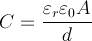where C is the capacitance, εr is the relative permittivity of the material, ε0 is the permittivity of vacuum, A is the area of the plates and d is the distance between the plates. It becomes clear that the larger εr is, the larger the resulting capacitance becomes. For example, air as a material has a relative permittivity of approximately 1, meaning that it acts as if the capacitor plates were placed in a vacuum. On the other hand, some polymers can have the relative permittivity of up to 100,000! Using such materials, it is possible to achieve the same capacitance in a much smaller volume, opening possibility for miniaturization.

Now let’s look at the dielectric strength. Consider an air capacitor, with the distance between the electrodes being 0.1mm. The dielectric strength of air is 3 megavolts per meter. This means that the maximum voltage that can be applied to this example capacitor is 300 volts under ideal conditions. The smaller the capacitor, the lower the maximum allowed voltage. All capacitors have maximum rated voltages which depend on the materials used, and exceeding these rated values could damage or destroy the capacitor.

4. Electric Charge

What is electric charge?

Electric charge is a fundamental physical property of matter. Electric charge can be positive or negative. Matter repels other matter of the same charge and attracts other matter having the opposite charge. The unit used for electric charge is a Coulomb [C]. While the exact nature of charge is still unknown at a fundamental level, it is generally accepted to represent a specific state of matter which cannot be explained at the current level of scientific knowledge.

Electric charge is quantized, meaning that charge can only have discrete values. An elementary charge is denoted as e, and approximately equals 1.602·10-19 C. The electron bears a charge of -e and it is a negatively charged particle. In contrast, a proton is a positively charged particle, bearing a charge of +e.

An intuitive way to understand the quantized nature of charge is to imagine an electrically neutral object as a box containing an equal number of protons (positive charges) and electrons (negative charges). Protons are fixed and cannot be taken out or added to the box. Since the number of protons and electrons is equal, the total sum of the electric charge inside the box is zero for electrically neutral objects.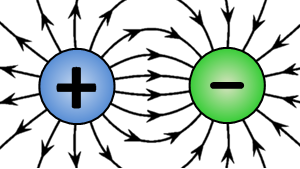In order to make the object negatively charged, the only way to do so is to somehow add more electrons into the box. As electrons are indivisible particles, it is only possible to add an integer number of electrons - one cannot add half an electron into the box. As a result, the total charge of the object is N times the charge of a single electron, which equals -e·N, where N is an integer number. Similarly, in order to make an object positively charged, it is necessary to remove N electrons from the box and the resulting total charge of the object is e·N in this case.

Net charge of an object

One way for an object to become electrically charged is to rub an object with another dissimilar object. This effect has been known since ancient times. For example, rubbing a glass rod with a piece of wool will result in the glass rod being positively charged, while the piece of wool will end up with a negative charge. This is known as the triboelectric effect, which uses friction to transfer free electrons from the glass rod to the wool piece. Since electrons are not created or destroyed in the process, the number of electrons leaving the glass rod is equal to the number of electrons which arrive at the piece of wool. As a result, the charge of the glass rod is equal in value to the charge of the piece of wool, but of opposite sign. Another way to charge an object is by applying an electric current using a power source.

Charging a capacitor

Capacitors are devices which are designed to store electric energy by accumulating electric charge on their electrodes. In a circuit, the charge leaves one electrode of the capacitor and arrives at the other electrode. Therefore, the amount of charge on one electrode is equal in value to the amount of charge on the other electrode, but of opposite sign. The more charge a capacitor stores, the more energy is stored on the capacitor, according to the formula: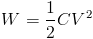where W is the energy stored, C is the capacitance and V is the voltage across the capacitor.

To understand the charging process, consider a completely discharged capacitor as the starting point. In order to charge a capacitor, a power source is connected to its terminals. The moment power is applied to the capacitor, the power source, for example a battery, begins to force electrons to move from one electrode of the capacitor to the other. As electrons start accumulating on one electrode, the voltage across the capacitor increases. Electrons keep accumulating on the electrode of the capacitor which is connected to the negative terminal of the battery until the voltage across the capacitor is equal to the voltage across the battery. This process is not linear - the voltage across the capacitor increases in an exponential manner. The equation for the voltage across the capacitor terminals as a function of time is: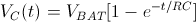where VC(t) is the voltage across the capacitor as a function of time, VBAT is the battery voltage, R is the series resistance in the circuit and C is the capacitance. The nature of R comes from the internal resistance of the battery combined with the ESR of the capacitor and any additional resistors wired in series between the battery and the capacitor.

5. Electric Field

What is an electric field?

An electric field is a special state that exists in the space surrounding an electrically charged particle. This special state affects all charged particles placed in the electric field. The true nature of electric fields, as well as the true nature of an electric charge is still unknown to scientists, but the effects of an electric field can be measured and predicted using known equations.

Just like a magnet creates an invisible magnetic field around it, which can be detected by placing a second magnet in its field and measuring the attractive or repulsive force acting on the magnets, electric charges create an electric field which can be detected by using a test charge. When a test charge is placed inside an electric field, an attractive or repulsive force acts upon it. This force is called the Coulomb force. In fact, magnetic and electric fields are not entirely separate phenomena. A magnetic field that changes with time creates - or “induces an electric field, while a moving electric field induces a magnetic field as a direct consequence of the movement. Because these two fields are so tightly connected, the magnetic and electric fields are combined into one, unified, electromagnetic field.

Electric field definition

The electric field can be defined as a vector field which describes the relationship between the charge of a test particle introduced in the field and the force exerted upon this charged test particle.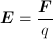Where E is the electric field, F is the force exerted on the test particle introduced into the field and q is the charge of the test particle. The unit for electric field is volts per meter [V·m-1] or newtons per coulomb [N·C-1].

The application of electric field in capacitors

Electromagnetism is a science which studies static and dynamic charges, electric and magnetic fields and their various effects. Capacitors are devices which store electrical potential energy using an electric field. As such, capacitors are governed by the rules of electromagnetism. This article will define and outline some of the terms which are needed to understand the workings of capacitors. In this article, it will be considered that the electric field is uniform in all points in space.

Electric potential energy

Electric potential energy is the potential energy of a charged particle in an electric field which results from the Coulomb force acting on the particle. It is defined as the negative of the amount of work needed to bring the particle from a reference point (often infinitely far away) to the point in space where the electric potential energy is measured. The unit for electric potential energy is a joule [J], the same unit used for the amount of work in physics.

Electric potential

Electric potential, also called the electric field potential, is the amount of electric potential energy that a charged particle would have at a certain point in space. The voltage, also called potential difference between two points in space is the difference of the electric potentials of those two points. The unit used for electric potential is a volt [V], named after the italian physicist Alessandro Volta. The same unit is used for voltage. The electric potential between two points in an uniform field is the negative of the field intensity difference between those two points.

Electric field strength

In a simple parallel-plate capacitor, a voltage applied between two conductive plates creates a uniform electric field between those plates. The electric field strength in a capacitor is directly proportional to the voltage applied and inversely proportional to the distance between the plates. This factor limits the maximum rated voltage of a capacitor, since the electric field strength must not exceed the breakdown field strength of the dielectric used in the capacitor. If the breakdown voltage is exceeded, an electrical arc is generated between the plates. This electric arc can destroy some types of capacitors instantly. The standard unit used for electric field strength is volts per meter [V·m-1].

Capacitance

Capacitance represents the ability of a body to store electrical charge. This ability is used in capacitors to store electrical energy by sustaining an electric field. When voltage is applied to a capacitor, a certain amount of positive electric charge (+q) accumulates on one plate of the capacitor, while an equal amount of negative electric charge (-q) accumulates on the other plate of the capacitor. It is defined as: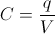where C is the capacitance, q is the amount of charge accumulated on the plates and V is the voltage across the two plates of the capacitor.

Capacitance is a function of the capacitor’s geometry. Factors such as the area of the plates, the distance between the plates and the dielectric constant of the dielectric used in the construction of the capacitor all influence the resulting capacitance. In a simple parallel plate, the electrical capacitance is directly proportional to the area of the plates and the dielectric constant, while it is inversely proportional to the distance between the plates. The unit used for capacitance is a Farad [F], named after Michael Faraday, who was the pioneer of research in electricity and magnetism.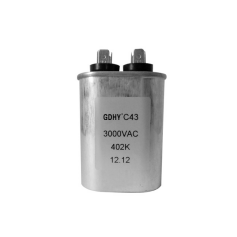Energy stored on a capacitor

Capacitors are devices which are used to store electrical energy in a circuit. The energy supplied to the capacitor is stored in the form of an electric field which is created between the plates of a capacitor. When the voltage is applied across a capacitor, a certain amount of charge accumulates on the plates. The energy stored on the capacitor is:where W is the energy stored, C is the capacitance and V is the voltage applied across the capacitor.

RECOMMENDED FOR YOU
GDHY Revolutionizing Capacitor Design to Enhance Performance and Efficiency in Small Welding and Quenching Equipment
Precautions for using film capacitors
Start capacitor in AC Induction Motor
How does metallized polyester film capacitors work in the application?
How to chose water cooled resonant capacitor ?
What is an axial film capacitor?
What is a plastic film capacitor?
IGBT Snubber Capacitors Selection Guide
What is a metallized polyester film capacitor?
What is a coupling capacitor and a bypass capacitor?
Get In Touch With Us
If you contact us now for more details, you can take samples for free. Our service team will get back to you with in 24 hours normally !